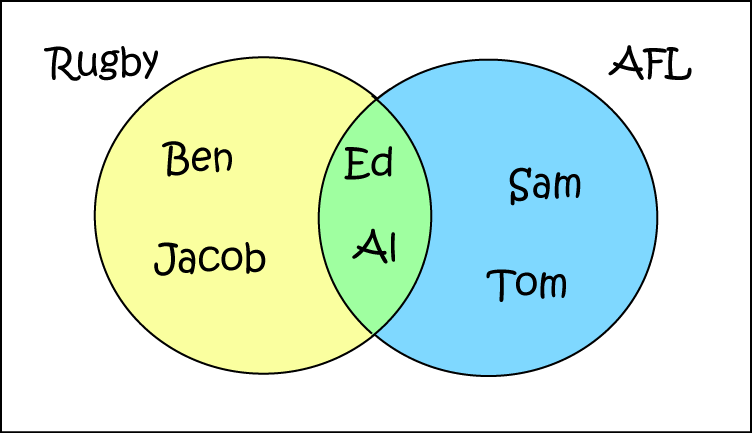# Definition of Intersection (sets)The intersection of two sets $A$ and $B$ is the set of all elements that are in both set $A$ and set $B$.

We use the symbol $\cap$ to say that we're taking an intersection.

For example, the Venn diagram in the picture shows the sets $\text{Rugby} = \{\text{Ben}, \text{Jacob}, \text{Ed}, \text{Al}\}$ and $\text{AFL} = \{\text{Ed}, \text{Al}, \text{Sam}, \text{Tom}\}$. Their intersection is $\text{Rugby} \cap \text{AFL} = \{\text{Ed},\text{Al}\}$. Ed and Al play both Rugby and AFL.

### Description

The aim of this dictionary is to provide definitions to common mathematical terms. Students learn a new math skill every week at school, sometimes just before they start a new skill, if they want to look at what a specific term means, this is where this dictionary will become handy and a go-to guide for a student.

### Audience

Year 1 to Year 12 students

### Learning Objectives

Learn common math terms starting with letter I

Author: Subject Coach
You must be logged in as Student to ask a Question.